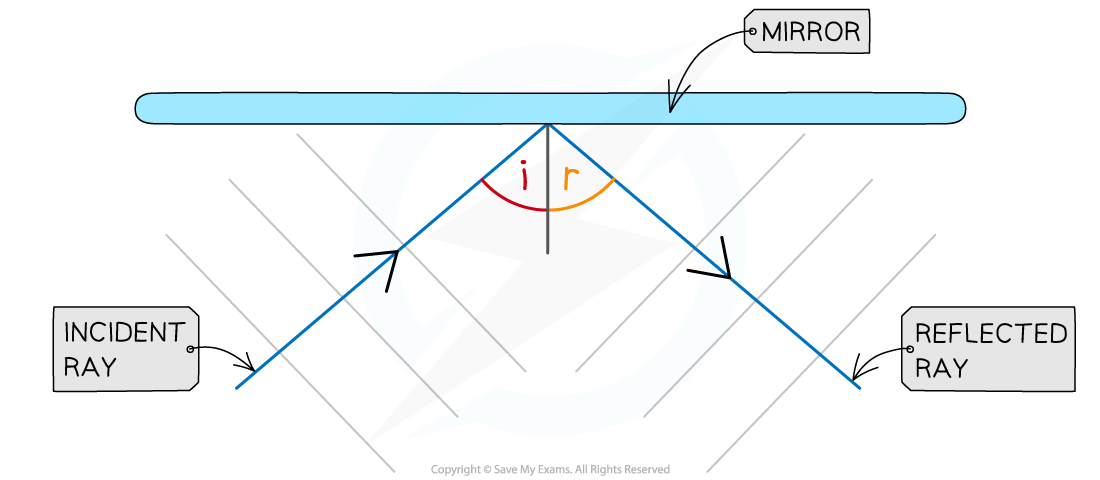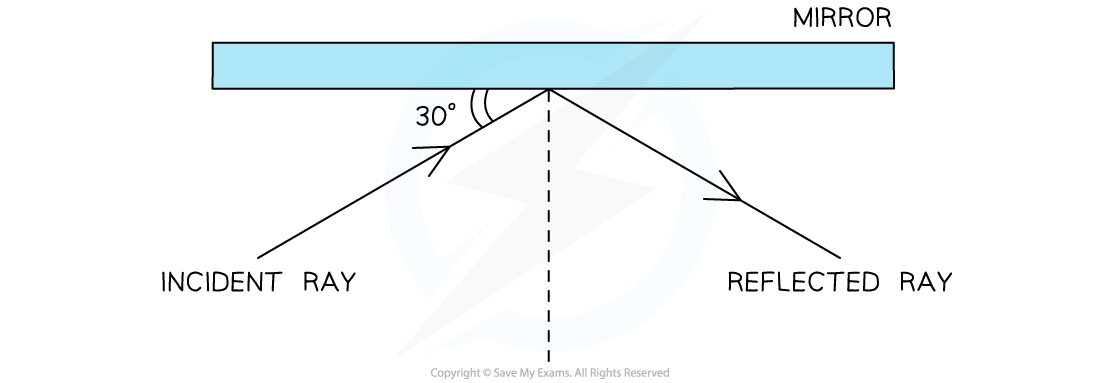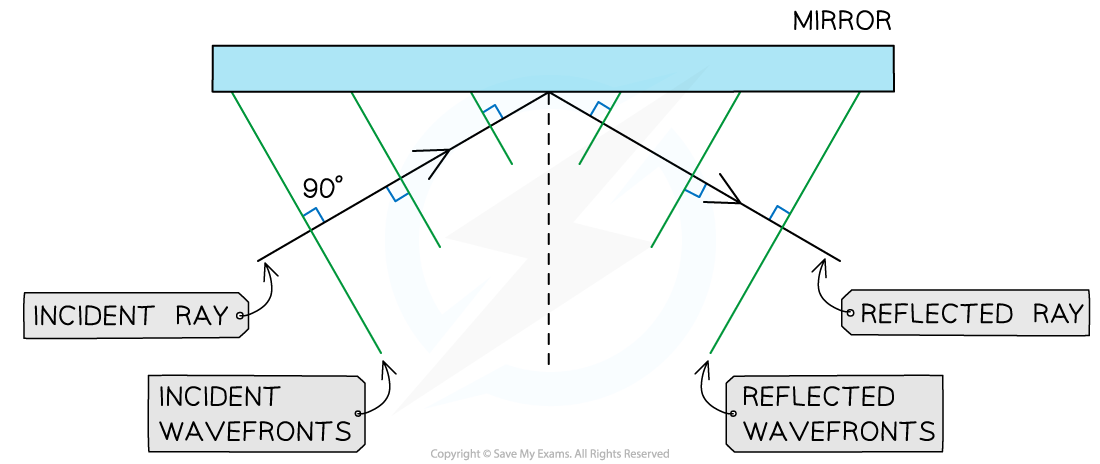# IB DP Physics: SL复习笔记4.4.2 Reflection

### Reflection

• When light hits a smooth plane surface, most of it gets reflected
• Very smooth reflective surfaces (e.g. mirrors) are known as specular plane reflectors
• For these surfaces, the law of reflection applies:

The angle of reflection is equal to the angle of incidence

i = r

• Where:
• The angle of incidence (i) is the angle between the incident ray and the normal
• The angle of reflection (r) is the angle between the reflected ray and the normalA light ray being reflected by a mirror

• The wavelength of the reflected ray is the same as that of the incident ray

#### Worked Example

A light ray is reflected by a mirror as shown in the diagram below.

a) State the angle of reflection

b) Add the incident and reflected wavefronts to the diagrama) State the angle of reflection

Step 1: Recall the definition of the angle of incidence (i)

• The angle of incidence is the angle between the incident ray and the normal
• This is not the 30° angle marked in the diagram

i = 90° – 30°

i = 60°Step 2: Recall the law of reflection

The angle of reflection is equal to the angle of incidence

Step 3: State the angle of reflection (r)

r = 60°

b)  Add the incident and reflected wavefronts to the diagram

Step 1: Recall that wavefronts and rays are perpendicular to each other

• Add at least three equally spaced wavefronts all perpendicular to the incident ray
• Add at least three equally spaced wavefronts all perpendicular to the reflected ray#### Exam Tip

When asked to complete or construct reflection ray diagrams, remember to add:

• arrows on rays to distinguish between incident and reflected rays
• labels to distinguish between incident and reflected wavefronts

In most cases, when dealing with light, you will just need to draw rays. However, you might still be required to draw wavefronts sometimes. Always use a ruler or a straight edge and a shape pencil for the ray diagrams.

Remember that the distance between each wavefront represents the wavelength of the wave. If you intend on the ray not changing wavelength (like in reflection) then make sure the incident and reflected wavefronts are all equally spaced by the same amount.